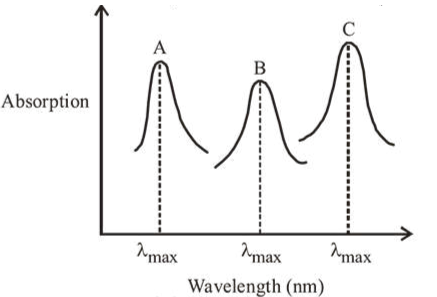# Simplified absorption spectra of three complexesQuestion:

Simplified absorption spectra of three complexes ((i), (ii) and (iii)) of $\mathrm{M}^{\mathrm{n}+}$ ion are provided below; their $\lambda_{\max }$ values are marked as A, B and C respectively. The correct match between the complexes and their $\lambda_{\max }$ values is :(i) $\left[\mathrm{M}(\mathrm{NCS})_{6}\right]^{(-6+n)}$

(ii) $\left[\mathrm{MF}_{6}\right]^{(-6+n)}$

(iii) $\left[\mathrm{M}\left(\mathrm{NH}_{3}\right)_{6}\right]^{n+}$

1. A-(iii), B-(i), C-(ii)

2. A-(ii), B-(i), C-(iii)

3. A-(ii), B-(iii), C-(i)

4. A-(i), B-(ii), C-(iii)

Correct Option: 1

Solution:

Stronger the ligand greater is splitting of $d$ orbitals and smaller will be wavelength of light absorbed.

According to spectrochemical series, the splitting power of ligands is $\mathrm{NH}_{3}>\mathrm{NC} \overline{\mathrm{S}}>\mathrm{F}^{-}$.Thus, order of wavelength of light absorbed is $\lambda_{\mathrm{NH}_{3}}<\lambda_{\mathrm{NCS}}<\lambda_{\mathrm{F}^{-}}$